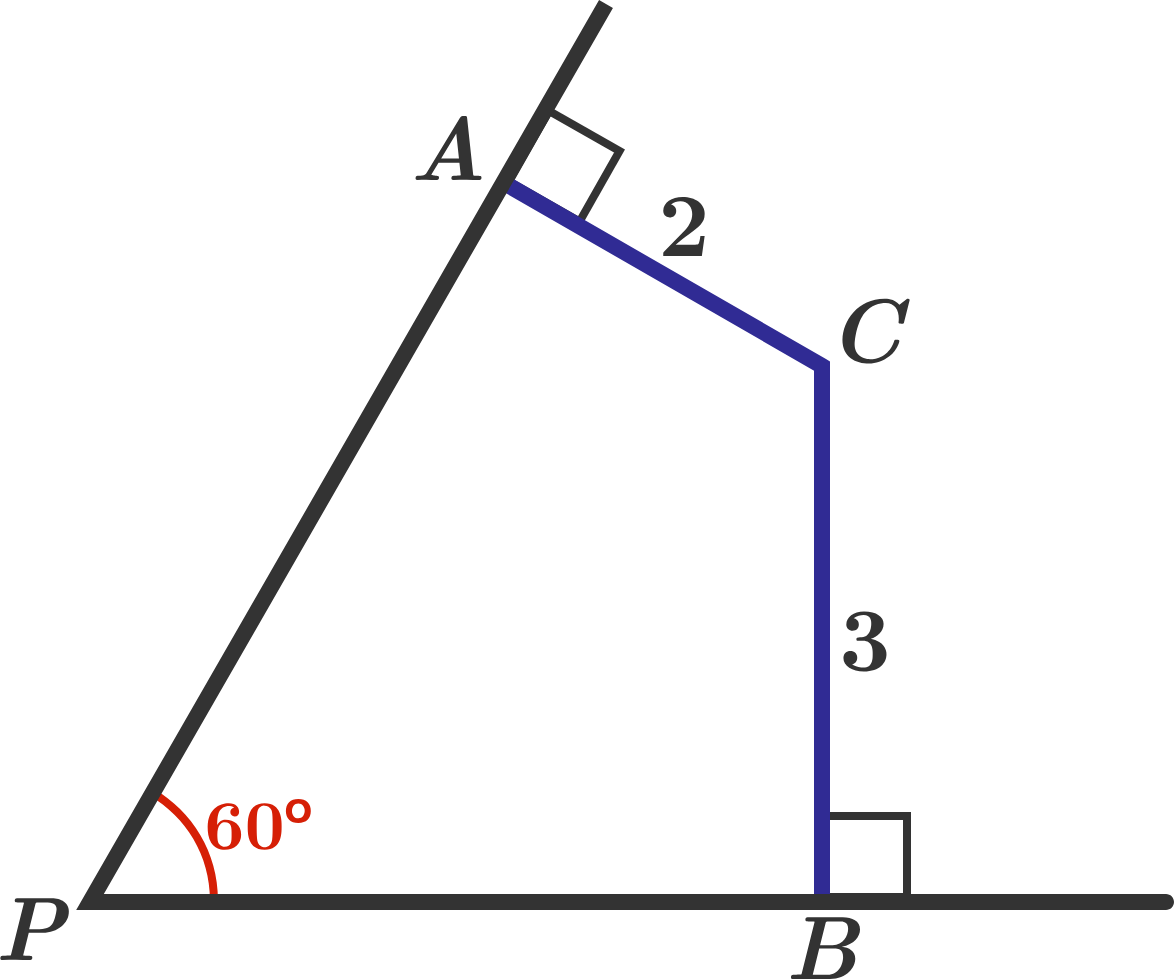# Just an Angle!

Geometry Level 3Find $PC.$

Note: This length can be expressed as $\sqrt{\dfrac{A}{B}}$, where $A,B$ are coprime positive integers. Submit the value of $A+B$ as your answer.

×